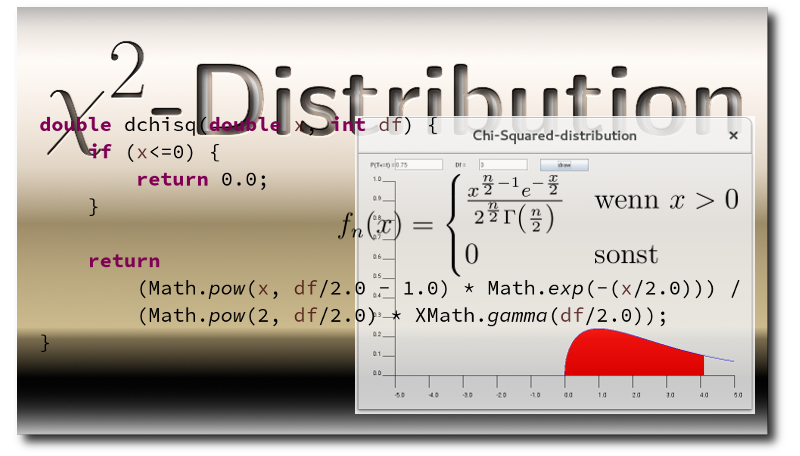# The Chi-Squared-Distribution - Compute and visualize in Java

in #programming2 years agoToday I add the chi-square distribution to my collection.

Since the same parameters are used here as for the student's t-distribution, the GUI does not need to be extended.

## Calculation of the distribution

``````    double dchisq(double x, int df) {
if (x<=0) {
return 0.0;
}

return
(Math.pow(x, df/2.0 - 1.0) * Math.exp(-(x/2.0))) /
(Math.pow(2, df/2.0) * XMath.gamma(df/2.0));
}

public double qchisq(double alpha, int df) {
double sum = 0;
double pos = 0;
double stepSize = 0.01;
while (sum<alpha) {
sum += dchisq(pos, df)*stepSize;
pos += stepSize;
}
return pos;
}
``````

Other distributions:

Sort:

Cool demonstration 👍